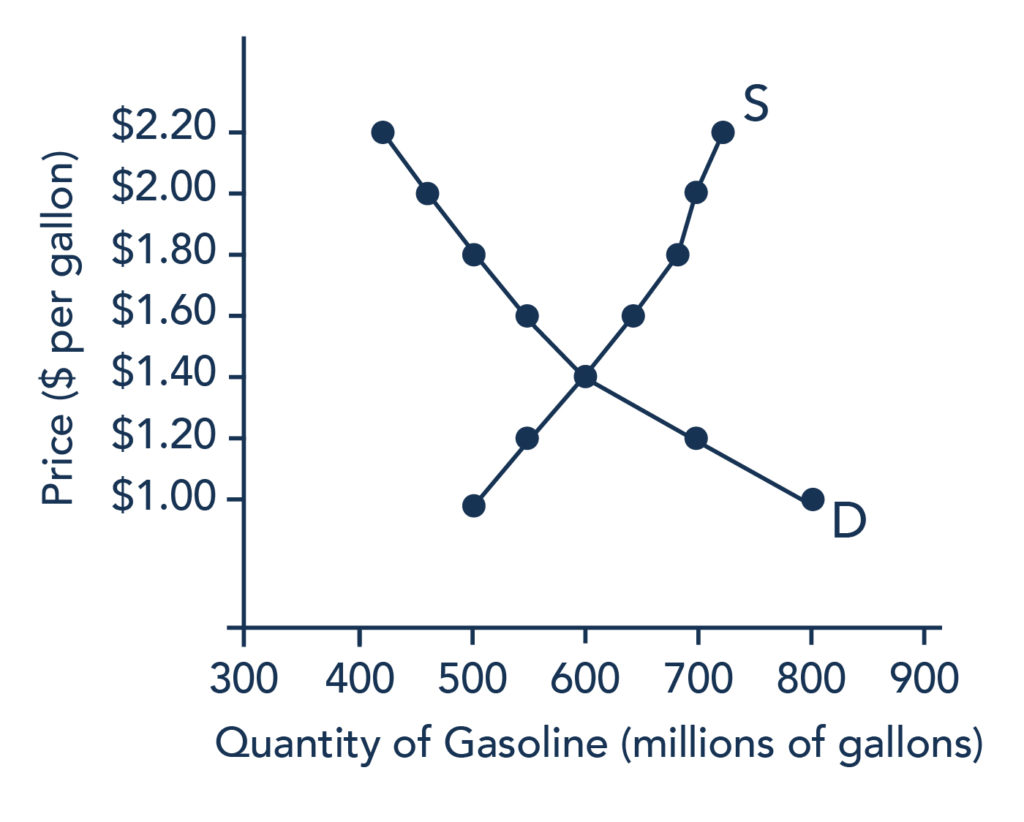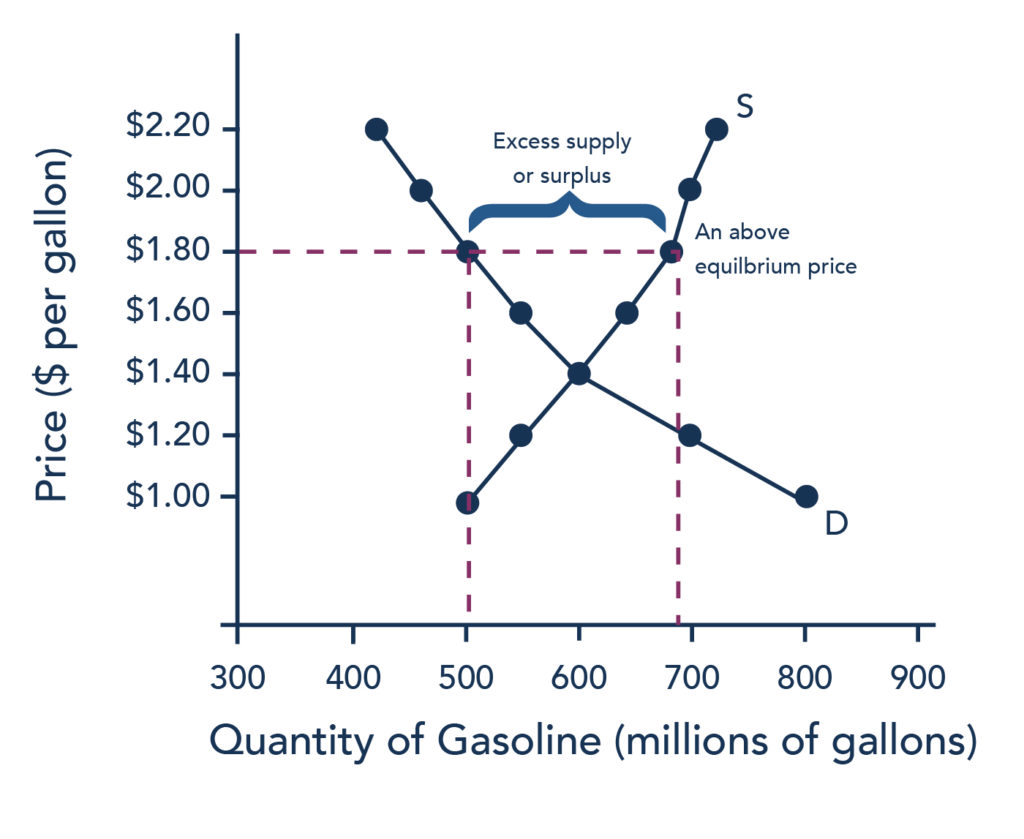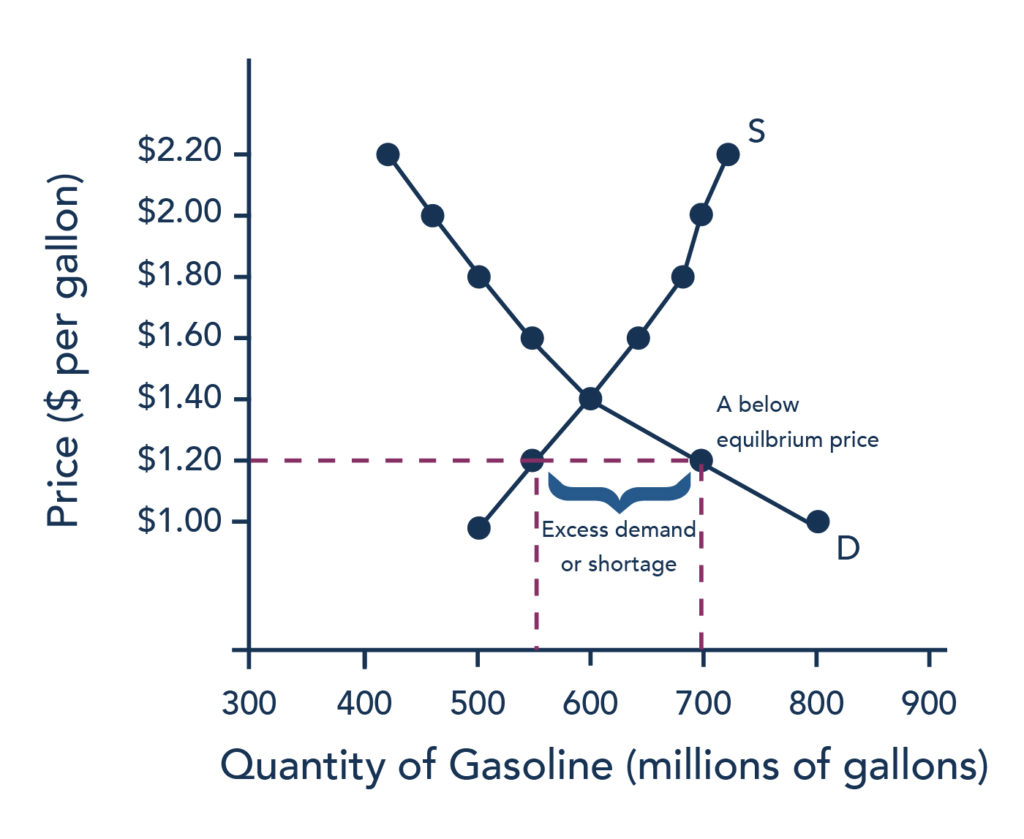## Surpluses and Shortages

### Learning Outcomes

• Explain surpluses and shortages

In order to understand market equilibrium, we need to start with the laws of demand and supply. Recall that the law of demand says that as price decreases, consumers demand a higher quantity. Similarly, the law of supply says that when price decreases, producers supply a lower quantity.

Because the graphs for demand and supply curves both have price on the vertical axis and quantity on the horizontal axis, the demand curve and supply curve for a particular good or service can appear on the same graph. Together, demand and supply determine the price and the quantity that will be bought and sold in a market. These relationships are shown as the demand and supply curves in Figure 1, which is based on the data in Table 1, below.Figure 1. The supply and demand curves for gasoline.

Table 1. Price, Quantity Demanded, and Quantity Supplied
Price (per gallon) Quantity demanded (millions of gallons) Quantity supplied (millions of gallons)
$1.00 800 500$1.20 700 550
$1.40 600 600$1.60 550 640
$1.80 500 680$2.00 460 700
$2.20 420 720 If you look at either Figure 1 or Table 1, you’ll see that at most prices, the amount that consumers want to buy (which we call the quantity demanded) is different from the amount that producers want to sell (which we call the quantity supplied). What does it mean when the quantity demanded and the quantity supplied aren’t the same? The answer is: a surplus or a shortage. ## Surplus or Excess Supply Let’s consider one scenario in which the amount that producers want to sell doesn’t match the amount that consumers want to buy. Consider our gasoline market example. Imagine that the price of a gallon of gasoline were$1.80 per gallon. This price is illustrated by the dashed horizontal line at the price of $1.80 per gallon in Figure 2, below.Figure 2. A price above equilibrium creates a surplus. At this price, the quantity demanded is 500 gallons, and the quantity of gasoline supplied is 680 gallons. You can also find these numbers in Table 1, above. Now, compare the quantity demanded and quantity supplied at this price. Quantity supplied (680) is greater than quantity demanded (500). Or, to put it in words, the amount that producers want to sell is greater than the amount that consumers want to buy. We call this a situation of excess supply (since Qs > Qd) or a surplus. Note that whenever we compare supply and demand, it’s in the context of a specific price—in this case,$1.80 per gallon.

With a surplus, gasoline accumulates at gas stations, in tanker trucks, in pipelines, and at oil refineries. This accumulation puts pressure on gasoline sellers. If a surplus remains unsold, then those firms involved in making and selling gasoline are not receiving enough cash to pay their workers and cover their expenses. In this situation, some firms will want to cut prices, because it is better to sell at a lower price than not to sell at all. Once some sellers start cutting prices, others will follow to avoid losing sales. These price reductions will, in turn, stimulate a higher quantity demanded.

How far will the price fall? Whenever there is a surplus, the price will drop until the surplus goes away. When the surplus is eliminated, the quantity supplied just equals the quantity demanded—that is, the amount that producers want to sell exactly equals the amount that consumers want to buy. We call this equilibrium, which means “balance.” In this case, the equilibrium occurs at a price of $1.40 per gallon and at a quantity of 600 gallons. You can see this in Figure 2 (and Figure 1), where the supply and demand curves cross. You can also find it in Table 1 (the numbers in bold). ### Practice Questions ## Shortage or Excess Demand Let’s return to our gasoline problem. Suppose that the price is$1.20 per gallon, as the dashed horizontal line at this price in Figure 3, below, shows. At this price, the quantity demanded is 700 gallons, and the quantity supplied is 550 gallons.Figure 3. A price below equilibrium creates a shortage.

Quantity supplied (550) is less than quantity demanded (700). Or, to put it in words, the amount that producers want to sell is less than the amount that consumers want to buy. We call this a situation of excess demand (since Qd > Qs) or a shortage.

In this situation, eager gasoline buyers mob the gas stations, only to find many stations running short of fuel. Oil companies and gas stations recognize that they have an opportunity to make higher profits by selling what gasoline they have at a higher price. These price increases will stimulate the quantity supplied and reduce the quantity demanded. As this occurs, the shortage will decrease. How far will the price rise? The price will rise until the shortage is eliminated, and the quantity supplied equals quantity demanded. In other words, the market will be in equilibrium again. As before, the equilibrium occurs at a price of \$1.40 per gallon and at a quantity of 600 gallons.

Generally, any time the price for a good is below the equilibrium level, incentives built into the structure of demand and supply will create pressures for the price to rise. Similarly, any time the price for a good is above the equilibrium level, similar pressures will generally cause the price to fall.

As you can see, the quantity supplied or quantity demanded in a free market will correct over time to restore balance, or equilibrium.

## Contribute!

Did you have an idea for improving this content? We’d love your input.